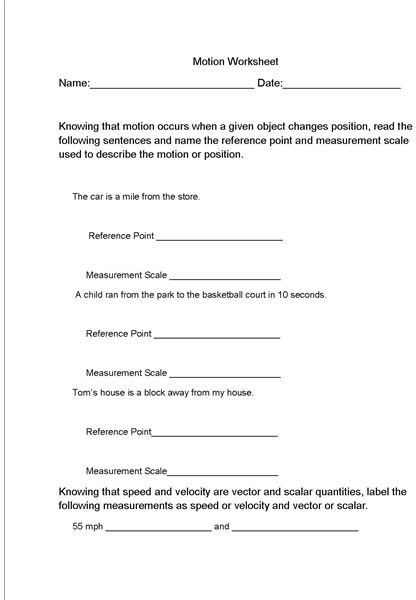Let’s Get Moving with This Lesson Plan on Motions

Teaching What Exactly is Motion?

Teachers, use this lesson plan to introduce the concept of motion as it concerns physical science by following the directions below.

1. Place two similar books on a table. Measure how far the books are from the edge of the table. Do this without students knowing it.
2. Ask a student to pick up a one of the books, but do not give the location of the object. Chances are the student will look puzzled as they have no idea where this object is.
3. Ask students why the book could not be picked up.
4. Explain to students that any motion first requires a location. The student could not pick up the book because no location was given.
5. Further explain to students that in science, location is referred to as position and requires a reference point as well as a measurement scale.
6. Again ask the student to pick up the book, this time giving the reference point of the object.
7. The student should again be confused and wonder which book to pick up. Ask why the student is confused.
8. Explain that the student needed more information, such as a measurement scale.
9. Ask the student to pick up the book and designate the reference point (table) as well as how far the book is from the edge of the table (measurement scale).
10. Explain to students what a measurement scale and reference point are and be sure they understand the meaning of each one.

Teaching Students to Measure Motion

Explain to students that motion is measured in scalar or vector quantities of speed, acceleration and velocity. Explain these terms to the students. Refer students to this study guide on the terms of motion to assist them in understanding terms like speed, acceleration and velocity.

Students are probably familiar with the term of speed, but may not realize that the velocity is simply the speed in a given direction. These terms will be reviewed further in future lesson plans.

Pass out the worksheet included with this lesson plan. Be sure that students understand the following terms so that they can complete the worksheet. This lesson plan and worksheet will help students understand and put into practice the various terms of motion.

Motion WorksheetReferences

• Student teaching experience.

This post is part of the series: Motion in Physical Science

This series on motion in physical science comes complete with worksheets and study guides for motion, speed, velocity and acceleration.